## 【数论】Fibonacci Sum – 20HDU多校1-5

### 传送门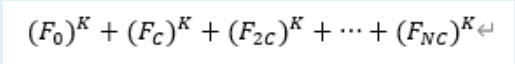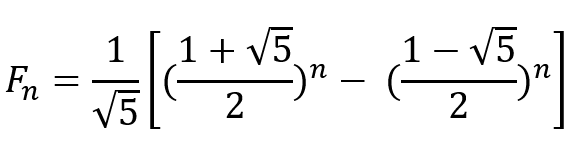383008016^2 ≡616991993^2≡5 (mod 10^9+9)

F_n=C(A^n-B^n)

F_{cn}^k=C^k(A^{cn}-B^{cn})^k

\sum\limits_{i=0}^n F_{ci}^k=C^k\sum\limits_{i=0}^n(A^{ci}-B^{ci})^k

\sum\limits_{i=0}^nF_{ci}^k= C^k\sum\limits_{i=0}^n \sum\limits_{r=0}^k \binom{k}{r}(A^{ci(k-r)}B^{cir}(-1)^r)

\sum\limits_{i=0}^nF_{ci}^k= C^k \sum\limits_{r=0}^k \binom{k}{r} (-1)^r \sum\limits_{i=0}^n (A^{ci(k-r)}B^{cir})• 注意本题从0累加到n实际上有n + 1项，求几何级数的时候需要注意。但是第0项等于0，所以也可以看作从1开始枚举，用通项的时候单独减去1。两种处理方法都可以。
• 第二个需要注意的点是公式仅适用于r =\not 1。如果r=1直接用r * n或者r*(n+1)即可。取决于上面一步是什么处理方法。

#pragma GCC optimize(2)
#include<bits/stdc++.h>
using namespace std;
typedef long long LL;
const int mod = 

## 转送门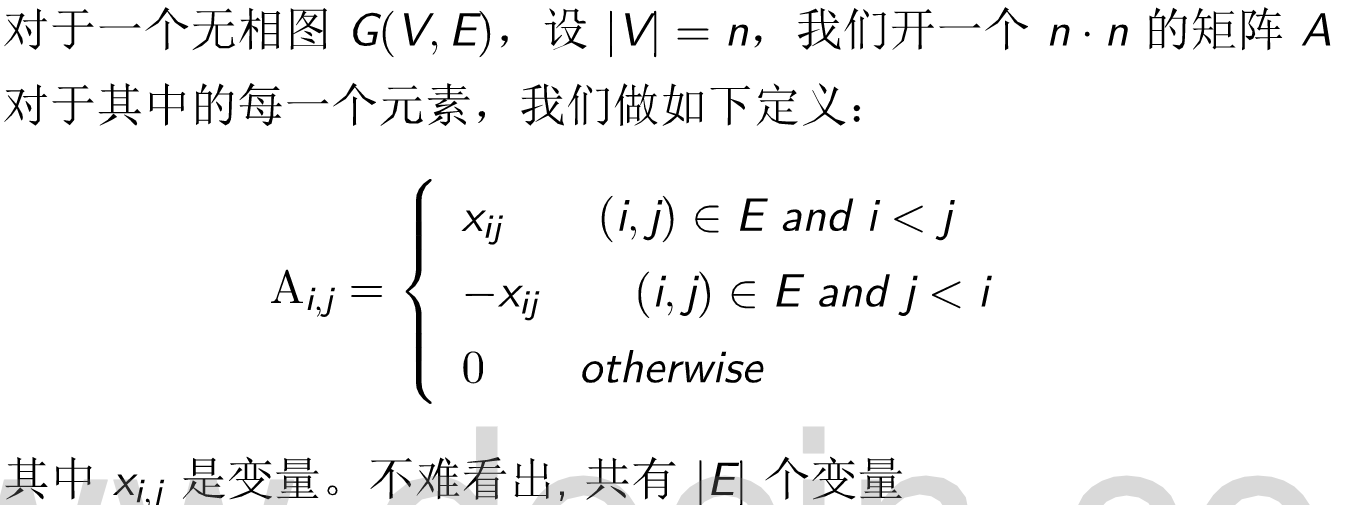#include<bits/stdc++.h>
using namespace std;
typedef long long ll;
mt19937 mrand(random_device{}()); // mrand是给定随机数种子的的大随机数对象
int rnd(int x) { return mrand() % x;}
const double eps = 1e-6;
const int maxn = 433;
ll a[maxn][maxn];
ll mod = 1e9 + 7;
ll ksm(ll n, ll k)
{
ll res = 1;
while(k)
{
if(k & 1) res = res * n % mod;
n = n * n % mod;
k >>= 1;
}
return res;


## 传送门

#include<bits/stdc++.h>
using namespace std;
const int maxn = (1 << 18) + 233;
typedef long long ll;
const ll mod = 1e9 + 7;
ll inv = 0;
ll ksm(ll n, ll k)
{
ll res = 1;
while(k)
{
if(k & 1) res = res * 

## 原题传送门

### 中文题面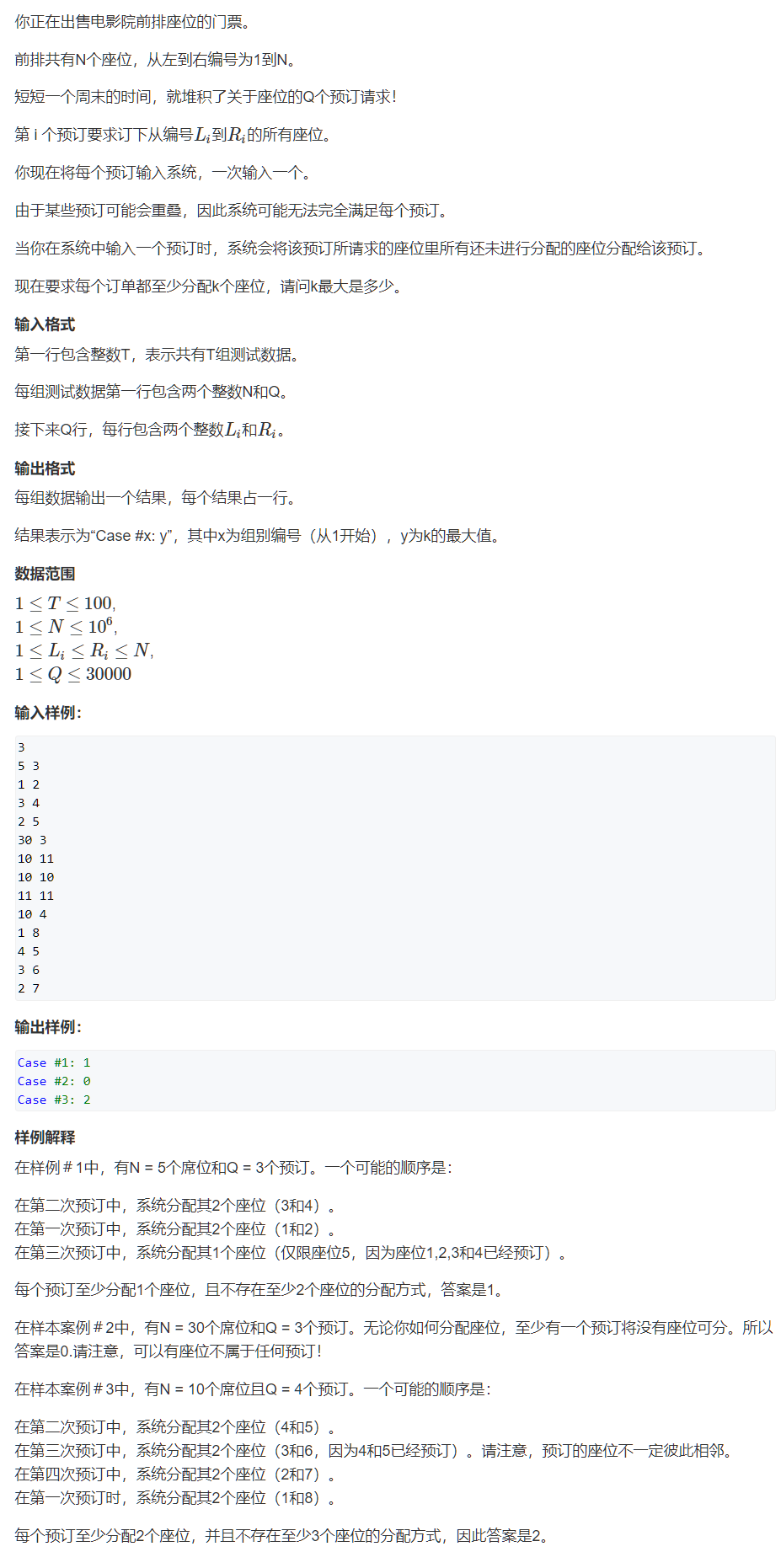1. 从最后一个人开始，枚举谁能得到最多的座位。假设是第X个询问。
2. 去掉第X个询问，再重复步骤一。
3. 重复以上步骤直到询问为空。从得到的答案里取一个最小值即是答案。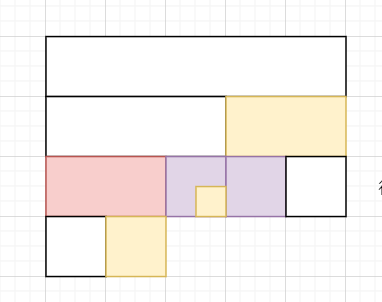#include<cstdio>
#include<algorithm>
#include<unordered_set>
#include<iostream>
#include<cstring>
using namespace std;
typedef pair<int, int> PII;
const int maxn = 1e6 + 23;
const int INF = 0x3f3f3f3f;
int n, q;
PII seg;
struct NodeQ {
int l, r;
int v;
}trQ[30300 * 4];
void buildQ(int u, int l, int r)
{
if (l == r) 

## 传送门

N和K都小于1E6

f[i][j] 表示前 i 天偷了 j 天懒。也就是说本来应该走i * k 个景点，但是只走了i * k – j个。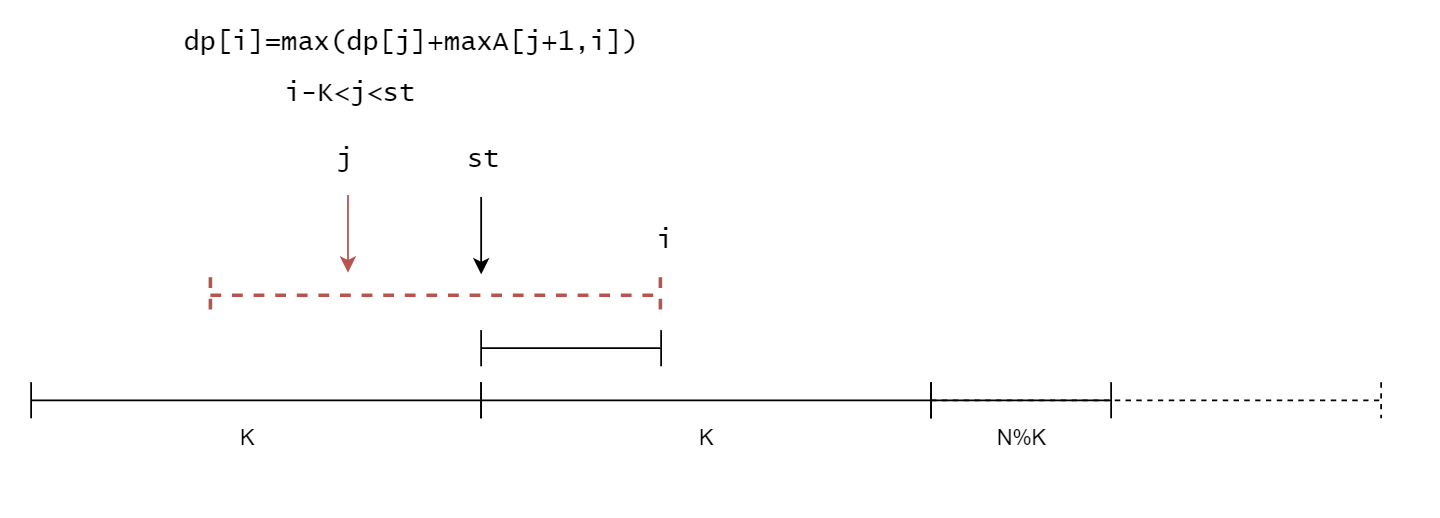#include<bits/stdc++.h>
using namespace std;
typedef long long ll;
const int maxn = 1e6 + 233;
ll c[maxn], 

## Z-algorithm(Z函数)模板

#include<bits/stdc++.h>
using namespace std;
const int maxn = 1e6 + 233;
char str[maxn];
int ne[maxn], z[maxn];
int main()
{
int T; scanf("%d", &T);
while(T--)
{
scanf("%s", str + 1);
int len = strlen(str + 1);
for(int i = 1; i <= len; i++) z[i] = 0;
z = len;
for(int i = 2, l = 0, r = 0; i <= len; i++)
{
if(i <= r) z[i] = min(z[i - l + 1], r - 

## 【线段树】区间不重复覆盖

### 传送门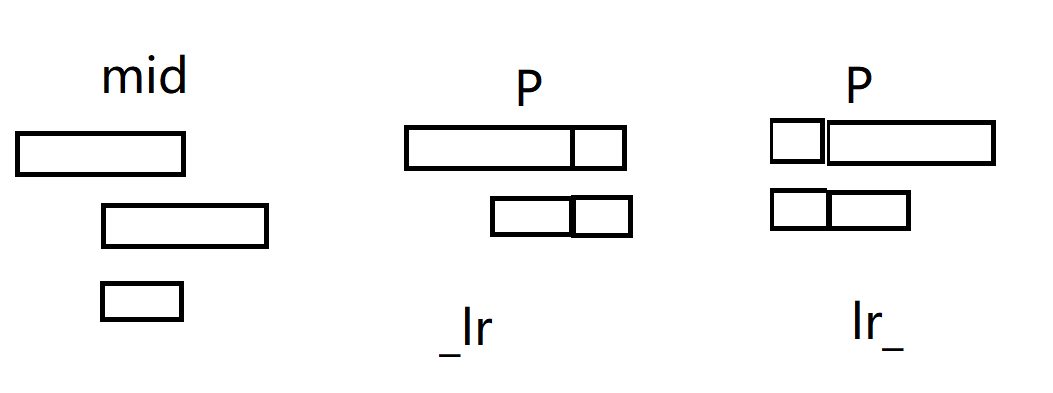“_LR” 代表区间左端点可以填为长度为1或者2的右端点，但右端点不超出。即可能向左超出
“LR_” 代表区间右端点可以填为长度为1或者2的左端点，但左端点不超出。即可能向右超出
“_LR_” 代表左右端点都有可能超出。
“L_R” 代表左右端点都不超出。

#include<bits/stdc++.h>
using namespace std;
const int maxn = 1e5 + 23;
struct Node{
int l, r;
int s, sl, sr, sn, lv, rv;
}tr[maxn * 4];
void pushup(Node &u, Node &l, Node &r)
{
u.lv = l.lv;
u.rv = r.rv;
int mv = l.rv & (1 << 1);
u.s = (l.s && r.s) || (l.sl && r.sr && mv);
u.sl = (l.s && r.sl) || (l.sl 

# 股票问题汇总

1. 不能进行多笔交易；买股票之前只能先把手上的股票卖出。一般此类题是dp。
2. 可以进行多笔交易；手里可以同时持有多只股票。一般要贪心。

#include<bits/stdc++.h>
using namespace std;
const int maxn = 1e5 + 233;
int w[maxn], f[maxn];
int main()
{
int n, k; scanf("%d%d", &n, &k);
for(int i = 1; i <= n; i++) scanf("%d", &w[i]);
memset(f, 0xcf, sizeof f);
for(int i = 0; i <= n; i++) f[i] = 0;
for(int i = 1; i <= n; i++)
{
for(int j = 1; j <= k; j++)
{
f[i][j] = max(f[i - 

## 【2017四川省赛/牛客】2017 Revenge【数论/指标】

### 传送门

#### 题意是说给2e6个数，每个数都小于2017，从中选取一些数字使得乘积模2017等于给定的r，问方案数%2输出

DP，f[i][ja[i]%2017]=f[i-1][j]+f[i-1][ja[i]]，因为答案只需要奇偶，所以考虑异或操作。

f[i][j+I[a[i]]]=f[i-1][j]+f[i-1][j+I[a[i]]]

f^=(f<<I[a[i]])^(f(2016-a[i]))

#include<bits/stdc++.h>
using namespace std;
bitset<2016> f;
int a, I;
int main()
{
int n, r;
int g = 1;
for(int i = 1; i <= 2016; i++)
{
g = g * 5 % 2017;
I[g] = i;
}
// I = 0;
while(~scanf("%d%d", &n, &r))
{
f.reset();
for(int i = 1; i <= n; i++) scanf("%d", &a[i]);
f.set(0);
for(int i = 1; i <= n; i++)
{
f 

## 【最短路/拆点】ACwing176

### 传送门

#include<bits/stdc++.h>
using namespace std;
const int maxn = 2e5 + 233;
int p[maxn], val[maxn], e[maxn], pre[maxn], h[maxn], cnt;
void add(int u, int v, int w)
{
e[cnt] = v; val[cnt] = w; pre[cnt] = h[u]; h[u] = cnt++;
}
struct Ver{
int a, b, c;
bool operator<(const Ver &W)const
{
return c > W.c;
}
};
int dis;
bool vis;
int dijkstra(int cost, int start, int end)
{
memset(dis, 0x3f, sizeof dis);
memset(vis,0, sizeof vis);
priority_queue<Ver> pq;
pq.push({start,